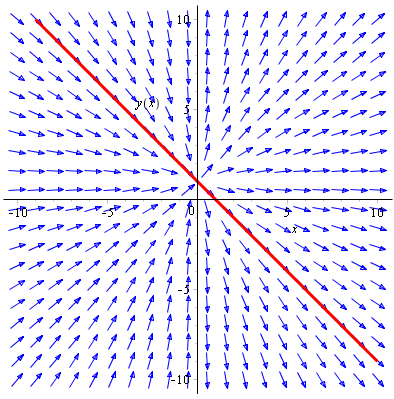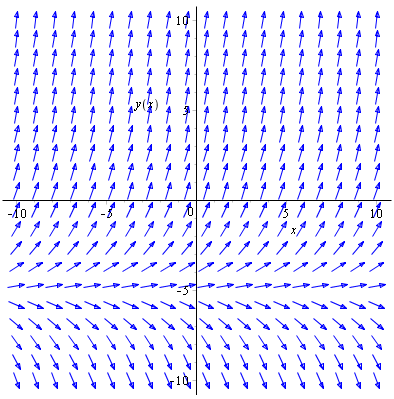# First order differential equations

## Introduction

Any relation between $y$, $\frac{dy}{dx}$, $\frac{d^{2}y}{dx^{2}}$, ... is called a differential equation.

If the highest order derivative in an equation is $\frac{d^{n}y}{dx^{n}}$ then the differential equation is order $n$.

When solving a differential equation we aim to find the base relationship between $y$ and $x$ in as simple terms as possible.

## Separation of variables

In C3 you learned that differential equations can sometimes be separated so that the solution can be found by integrating

$$\frac{dy}{dx} = \frac{f(x)}{g(y)}$$

$$\Rightarrow \int g(y) \mathop{dy} = \int f(x) \mathop{dx}$$

This yields an integral on either side with a constant of integration. The constants of integration on either side can be merged into a single arbitrary constant.

The presence of an arbitrary constant means you have gained a general solution. In particular situations you may already know something about the solution function. Maybe you know that at $x=0$, $y=0$. That is a boundary condition which you sub in to the general solution to find the particular solution.

### Example

Q) Solve $\frac{dy}{dx} = \frac{y-1}{x}$, given that $y=0$ when $x=1$.

A) By rearranging we can separate variables

$$\frac{dy}{dx} = \frac{y-1}{x}$$

$$\frac{1}{y-1}\frac{dy}{dx} = \frac{1}{x}$$

$$\Rightarrow \int \frac{1}{y-1} \mathop{dy} = \int \frac{1}{x} \mathop{dx}$$

So we come up with a single arbitrary constant of integration $C$

$$\ln\left|y-1\right| = \ln\left|x\right| + C$$

$$e^{\ln\left|y-1\right|} = e^{\ln\left|x\right| + C}$$

Let $a = e^{C}$, so

$$y-1 = ax$$

$$\Rightarrow y = ax+1$$

That is the general solution. But we were told the boundary condition $y\left(1\right)=0$ so

$$0 = a +1 \Rightarrow a = -1$$

Therefore the particular solution is $y = 1-x$.

## Family of solution curves

A consequence of the arbitrary constant in general solutions is that you can plot a family of solutions curves for many values of the arbitrary constant.

In the last example the general solution was $y = ax+1$. So the family of solutions curves is a load of functions all with different values of the constant $a$. Looking at the general solution, all the curves will be straight lines passing through $(0,1)$. The only thing to vary will be the gradient of the straight lines.

Here's what they look like. The highlighted line is the particular solution $y = 1-x$ arising from the boundary condition $y\left(1\right)=0$.Each arrow points in the direction of the gradient $\frac{dy}{dx}$ at that point in the 2D plane. The original differential equation $\frac{dy}{dx} = \frac{y-1}{x}$ shows that $\frac{dy}{dx}$ varies with both $x$ and $y$, and so the gradient is different depending on where you are in the 2D plane.

### Example

Q) Find the general solution of the differential equation $\frac{dy}{dx} = \frac{y+5}{2}$ and sketch the family of solutions curves.

A) Rearranging and integrating

$$\frac{dy}{dx} = \frac{y+5}{2}$$

$$\int \frac{2}{y+5} \mathop{dy} = \int dx$$

$$\Rightarrow 2\ln\left|y+5 \right| = x + C$$

$$\ln\left|y+5 \right| = \frac{x + C}{2}$$

$$\Rightarrow y+5 = e^{\frac{x + C}{2}}$$

Let $a = e^{\frac{C}{2}}$, so

$$y = ae^{\frac{x}{2}}-5$$

And the family of solutions curvesThe original differential equation $\frac{dy}{dx} = \frac{y+5}{2}$ shows that $\frac{dy}{dx}$ only varies with $y$ and not $x$. That means that the gradient at each point only changes if you go upwards and downwards. The gradients stay constant as you move horizontally from point to point.

## First order exact differential equations

Take the differential equation

$$y + x\frac{dy}{dx} = \sin x$$

The variables can't be separated, but notice that the left hand side is equal to $\frac{d}{dx}(xy)$ from the product rule. Then the differential equation becomes

$$\frac{d}{dx}(xy) = \sin x$$

Integrate both sides with respect to $x$ to get the general solution

$$xy = -\cos x + C$$

Differential equations of this form where one side is the exact derivative of a product, and the other can be integrated with respect to the independent variable ($x$ in this case) is an first order exact differential equation.

## First order linear differential equations

A first order linear differential equation takes the form

$$\frac{dy}{dx} + Py = Q$$

where $P$ and $Q$ can be functions of $x$ or constants. We can make the differential equation exact by multiplying both sides by an integrating factor, a function of $x$ gained by using $P$.

Specifically the integrating factor is equal to

$$e^{\int P \mathop{dx}}$$

Suppose there exists a function $f(x)$ such that the linear differential equation $$\frac{dy}{dx} + Py = Q$$ where $P$ and $Q$ are either functions of $x$ or constants, is made an exact differential equation by multiplying through by $f(x)$. In other words $$f(x) \frac{dy}{dx} + f(x)Py \equiv \frac{d}{dx}\left[f(x)y\right]$$ But by the product rule $$\frac{d}{dx}\left[f(x)y\right] = f(x) \frac{dy}{dx} + f'(x)y$$ By comparing the two, $$f(x)P = f'(x)$$ Divide both sides by $f(x)$ and integrate with respect to $x$ $$\int P \mathop{dx} = \int \frac{f'(x)}{f(x)}\mathop{dx} = \ln\left(f(x)\right)$$ Therefore $$f(x) = e^{\int P \mathop{dx}}$$

### Example

Q) Find the general solution of $\frac{dy}{dx} + \frac{y}{x} = 2$.

A) $P(x) = \frac{1}{x}$ and $Q(x) = 2$. Therefore the integrating factor is

$$e^{\int \frac{1}{x} \mathop{dx}} = e^{\ln x } = x$$

Multiply both sides by the integrating factor

$$\frac{dy}{dx}x + y = 2x$$

The left hand side is equal to $\frac{d}{dx}\left(xy\right)$, so integrate both sides with respect to $x$

$$\frac{d}{dx}\left(xy\right) = 2x$$

$$\Rightarrow xy = \int 2x \mathop{dx} = x^{2} + C$$

Therefore the general solution is

$$xy = x^{2} + C$$

Where $C$ is an arbitrary constant.